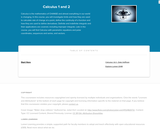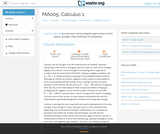# 42 Results

View
Selected filters:
• Calculus & BeyondUnrestricted Use
CC BY
Rating

Applied Calculus instructs students in the differential and integral calculus of elementary functions with an emphasis on applications to business, social and life science. Different from a traditional calculus course for engineering, science and math majors, this course does not use trigonometry, nor does it focus on mathematical proofs as an instructional method.

Subject:
Calculus & Beyond
Material Type:
Full Course
Textbook
Provider:
Lumen Learning
Provider Set:
Candela Courseware
Author:
Dale Hoffman
David Lippman
Shana Calaway
06/11/2019Unrestricted Use
CC BY
Rating

This course provides an introduction to applied concepts in Calculus that are relevant to the managerial, life, and social sciences. Students should have a firm grasp of the concept of functions to succeed in this course. Topics covered include derivatives of basic functions and how they can be used to optimize quantities such as profit and revenues, as well as integrals of basic functions and how they can be used to describe the total change in a quantity over time.

Subject:
Calculus & Beyond
Material Type:
Full Course
Textbook
Provider:
Lumen Learning
Provider Set:
Candela Courseware
06/11/2019Unrestricted Use
CC BY
Rating

Calculus is the mathematics of CHANGE and almost everything in our world is changing. In this course, you will investigate limits and how they are used to calculate rate of change at a point, define the continuity of a function and how they are used to define derivatives. Definite and indefinite integrals and their applications are covered, including improper integrals. Late in the course, you will find Calculus with parametric equations and polar coordinates, sequences and series, and vectors.

Subject:
Calculus & Beyond
Material Type:
Full Course
Textbook
Provider:
Lumen Learning
Provider Set:
Candela Courseware
Author:
Dale Hoffman
David Lippman
Jeff Eldridge
Melonie Rasmussen
06/11/2019Conditional Remix & Share Permitted
CC BY-NC-SA
Rating

This series of videos focusing on calculus covers sample questions from the A.P, Calculus AB and AC exams (both multiple choice and free answer).

Subject:
Calculus & Beyond
Material Type:
Lecture
Provider:
Provider Set:
Author:
Salman Khan
05/16/2019Conditional Remix & Share Permitted
CC BY-NC-SA
Rating

The Calculus BC AP exam is a super set of the AB exam. It covers everything in AB as well as some of the more advanced topics in integration, sequences and function approximation. This tutorial is great practice for anyone looking to test their calculus mettle!

Subject:
Calculus & Beyond
Material Type:
Lecture
Provider:
Provider Set:
Author:
Salman Khan
05/16/2019Conditional Remix & Share Permitted
CC BY-NC-SA
Rating

This series of videos focusing on calculus covers minima, maxima, and critical points, rates of change, optimization, rates of change, L'Hopital's Rule, mean value theorem.

Subject:
Calculus & Beyond
Material Type:
Lecture
Provider:
Provider Set:
Author:
Salman Khan
05/16/2019Conditional Remix & Share Permitted
CC BY-NC-SA
Rating

A single definite integral can be used to find the area under a curve. with double integrals, we can start thinking about the volume under a surface!

Subject:
Calculus & Beyond
Material Type:
Lecture
Provider:
Provider Set:
Author:
Salman Khan
05/16/2019Conditional Remix & Share Permitted
CC BY-NC-SA
Rating

This is about as many integrals we can use before our brains explode. Now we can sum variable quantities in three-dimensions (what is the mass of a 3-D wacky object that has variable mass)!

Subject:
Calculus & Beyond
Material Type:
Lecture
Provider:
Provider Set:
Author:
Salman Khan
05/16/2019Unrestricted Use
CC BY
Rating

This course begins with a review of algebra specifically designed to help and prepare the student for the study of calculus, and continues with discussion of functions, graphs, limits, continuity, and derivatives. The appendix provides a large collection of reference facts, geometry, and trigonometry that will assist in solving calculus problems long after the course is over. Upon successful completion of this course, the student will be able to: calculate or estimate limits of functions given by formulas, graphs, or tables by using properties of limits and LĺÎĺ_ĺĚĺ_hopitalĺÎĺ_ĺĚĺ_s Rule; state whether a function given by a graph or formula is continuous or differentiable at a given point or on a given interval and justify the answer; calculate average and instantaneous rates of change in context, and state the meaning and units of the derivative for functions given graphically; calculate derivatives of polynomial, rational, common transcendental functions, and implicitly defined functions; apply the ideas and techniques of derivatives to solve maximum and minimum problems and related rate problems, and calculate slopes and rates for function given as parametric equations; find extreme values of modeling functions given by formulas or graphs; predict, construct, and interpret the shapes of graphs; solve equations using NewtonĺÎĺ_ĺĚĺ_s Method; find linear approximations to functions using differentials; festate in words the meanings of the solutions to applied problems, attaching the appropriate units to an answer; state which parts of a mathematical statement are assumptions, such as hypotheses, and which parts are conclusions. This free course may be completed online at any time. It has been developed through a partnership with the Washington State Board for Community and Technical Colleges; the Saylor Foundation has modified some WSBCTC materials. (Mathematics 005)

Subject:
Calculus & Beyond
Material Type:
Assessment
Full Course
Syllabus
Textbook
Provider:
The Saylor Foundation
06/11/2019Conditional Remix & Share Permitted
CC BY-NC-SA
Rating

This series of videos focusing on calculus covers indefinite integral as anti-derivative, definite integral as area under a curve, integration by parts, u-substitution, trig substitution.

Subject:
Calculus & Beyond
Material Type:
Lecture
Provider:
Provider Set:
Author:
Salman Khan
05/16/2019Conditional Remix & Share Permitted
CC BY-NC-SA
Rating

This 9-minute video lesson provides an introduction to L'Hopital's Rule. [Calculus playlist: Lesson 36 of 156]

Subject:
Calculus & Beyond
Material Type:
Lecture
Provider:
Provider Set:
Author:
Salman Khan
02/20/2011Conditional Remix & Share Permitted
CC BY-NC-SA
Rating

This series of videos focusing on calculus covers limit introduction, squeeze theorem, and epsilon-delta definition of limits.

Subject:
Calculus & Beyond
Material Type:
Lecture
Provider:
Provider Set:
Author:
Salman Khan
05/16/2019Conditional Remix & Share Permitted
CC BY-NC-SA
Rating

Limits are the core tool that we build upon for calculus. Many times, a function can be undefined at a point, but we can think about what the function "approaches" as it gets closer and closer to that point (this is the "limit"). Other times, the function may be defined at a point, but it may approach a different limit. There are many, many times where the function value is the same as the limit at a point. Either way, this is a powerful tool as we start thinking about slope of a tangent line to a curve.

Subject:
Calculus & Beyond
Material Type:
Lecture
Provider:
Provider Set:
Author:
Salman Khan
05/16/2019Conditional Remix & Share Permitted
CC BY-NC-SA
Rating

This tutorial covers much of the same material as the "Limits" tutorial, but does it with Sal's original "old school" videos. The sound, resolution or handwriting isn't as good, but some people find them more charming.

Subject:
Calculus & Beyond
Material Type:
Lecture
Provider:
Provider Set:
Author:
Salman Khan
05/16/2019Conditional Remix & Share Permitted
CC BY-NC-SA
Rating

This series of videos focusing on calculus covers line integral of scalar and vector-valued functions, Green's Theorem and 2-D Divergence Teorem.

Subject:
Calculus & Beyond
Material Type:
Lecture
Provider:
Provider Set:
Author:
Salman Khan
05/16/2019Conditional Remix & Share Permitted
CC BY-NC-SA
Rating

It is sometimes easier to take a double integral (a particular double integral as we'll see) over a region and sometimes easier to take a line integral around the boundary. Green's theorem draws the connection between the two so we can go back and forth. This tutorial proves Green's theorem and then gives a few examples of using it. If you can take line integrals through vector fields, you're ready for Mr. Green.

Subject:
Calculus & Beyond
Material Type:
Lecture
Provider:
Provider Set:
Author:
Salman Khan
05/16/2019Conditional Remix & Share Permitted
CC BY-NC-SA
Rating

With traditional integrals, our "path" was straight and linear (most of the time, we traversed the x-axis). Now we can explore taking integrals over any line or curve (called line integrals).

Subject:
Calculus & Beyond
Material Type:
Lecture
Provider:
Provider Set:
Author:
Salman Khan
05/16/2019Conditional Remix & Share Permitted
CC BY-NC-SA
Rating

You've done some work with line integral with scalar functions and you know something about parameterizing position-vector valued functions. In that case, welcome! You are now ready to explore a core tool math and physics: the line integral for vector fields. Need to know the work done as a mass is moved through a gravitational field. No sweat with line integrals.

Subject:
Calculus & Beyond
Material Type:
Lecture
Provider:
Provider Set:
Author:
Salman Khan
05/16/2019Conditional Remix & Share Permitted
CC BY-NC-SA
Rating

This 17-minute video lesson looks at the iIntuition behind the Mean Value Theorem. [Calculus playlist: Lesson 55 of 156]

Subject:
Calculus & Beyond
Material Type:
Lecture
Provider:
Provider Set:
Author:
Salman Khan
02/20/2011Conditional Remix & Share Permitted
CC BY-NC-SA
Rating

Let's jump out of that boring (okay, it wasn't THAT boring) 2-D world into the exciting 3-D world that we all live and breath in. Instead of functions of x that can be visualized as lines, we can have functions of x and y that can be visualized as surfaces. But does the idea of a derivative still make sense? Of course it does! As long as you specify what direction you're going in. Welcome to the world of partial derivatives!

Subject:
Calculus & Beyond
Material Type:
Lecture
Provider: